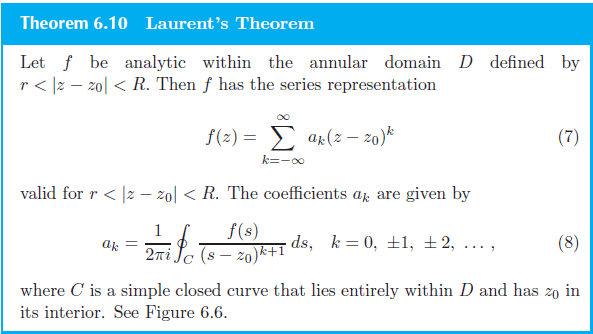# Laurent expansion of ##ze^{1/z}##

## Homework Statement

Find a Laurent series of ##f(z)=ze^{1/z}## in powers of ##z-1##. Is there an easier way to go about this as this is not a typical expansion I see on textbooks. It seems that my incomplete solution is too complicated. Please help, exam is in two days and I am working on past exams. Worked out solutions are welcome, also. P.S. it may seem like I'm cramming, but it's not under my control -## Homework Equations## The Attempt at a Solution

Since ##f(z)## is entire, then it is analytic in any annular domain. So let's pick the domain ##\frac{1}{2} \lt \vert z-1\vert \lt 3##. By Laurent's theorem the coefficients
\begin{align}a_k=\frac{1}{2\pi i}\int_{c}\frac{(1+e^{it})e^{\frac{1}{1+e^{it}}}}{e^{it\cdot k}}\end{align}
where ##C## is the contour ##z(t)=1+e^{it}## and hence, ##dz=ie^{it}dt##. Note that the contour ##C## is within our selected annular domain as it should be according to the theorem.

#### Attachments

Last edited:

Homework Helper
Gold Member
I think I see a trick to this one: ## f'(z)=(z-1)f(z) ##, and if you do the algebra, and I think you can show ## f^n(z)=(z-1)f^{n-1}+(n-1) f^{n-2}(z) ##. ## \\ ##That would make it suitable for a Taylor expansion about ## z=1 ##. Note that ##f^n(1)=(n-1)f^{n-2}(1) ##. ## \\ ## It looks like the odd number derivatives are zero and the even ones are ## (n-1)!! =(n-1)(n-3)(n-5)....(1) ##. (I think I have the correct definition of n!!=Edit: yes I googled it: see http://mathworld.wolfram.com/DoubleFactorial.html) And hopefully I don't get dinged for supplying a solution to this, because other than this approach, I wouldn't know what to do with this one.Last edited:
f′(z)=(z−1)f(z)
I don't see why this is true. I got \begin{align}f'(z)=e^{1/z}-\frac{e^{1/z}}{z}\end{align}
But ##(z-1)f(z)=(z-1)ze^{1/z}=z^2e^{1/z}-ze^{1/z}##

Homework Helper
Gold Member
I made an algebraic error here: ## f'(z)=\frac{(z-1)}{z^2} f(z) ##. Oh well, back to the drawing board.

•Terrell
Homework Helper
Gold Member
I think a similar method might still be possible: Using ## f'(z)=f(z)g(z) ## where ## g(z)=\frac{1}{z}-\frac{1}{z^2} ##, so that ## f'=fg ##, ## f''=f'g+fg' ##, etc, I think you should be able to get some kind of expression for ## f^n(z) ##.

•Terrell
I think a similar method might still be possible: Using f′(z)=f(z)g(z)f′(z)=f(z)g(z) f'(z)=f(z)g(z) where g(z)=1z−1z2g(z)=1z−1z2 g(z)=\frac{1}{z}-\frac{1}{z^2} , so that f′=fgf′=fg f'=fg , f′′=f′g+fg′f″=f′g+fg′ f''=f'g+fg' , etc, I think you should be able to get some kind of expression for fn(z)fn(z) f^n(z) .
Is there a standard general approach you are trying to follow? Thanks!!

•Homework Helper
Gold Member
Is there a standard general approach you are trying to follow? Thanks!!
I am just trying to find something that works. Had I not made a simple algebraic error, the first solution that I had would have been a good one. Upon further inspection, I'm not having any luck with anything that works...

•Terrell
I am just trying to find something that works. Had I not made a simple algebraic error, the first solution that I had would have been a good one. Upon further inspection, I'm not having any luck with anything that works...
I find it hard to believe they put this on a past exam. It's suppose to be a test of understanding, not puzzles. I'll try my hand at this again later, I'm working on other problems for the moment. Thank you!!

Homework Helper
Gold Member
I find it hard to believe they put this on a past exam. It's suppose to be a test of understanding, not puzzles. I'll try my hand at this again later, I'm working on other problems for the moment. Thank you!!
I hope the people who designed the problem didn't make the same mistake that I did in thinking there was a simple solution. It's unlikely, but certainly quite possible.•Terrell
I hope the people who designed the problem didn't make the same mistake that I did in thinking there was a simple solution. It's unlikely, but certainly quite possible.I would be outrage! These are comprehensive exams and they don't get returned to us.•Homework Helper
Gold Member
@Orodruin You have much more math expertise. Does this one have a simple solution that we are missing?

•Terrell
stevendaryl
Staff Emeritus
Here's my short-cut way to an answer:

##f(z) = z e^\frac{1}{z}## has a singularity only at ##z=0##. Which means that it doesn't have any singularity at ##z=1##. If it doesn't have a pole at ##z=1##, that means that it can be expanded in an ordinary Taylor series, using just positive powers of ##z-1##.

Given that, I think I would just let ##z = u+1## and then our function becomes ##f(u) = (1+u) e^{\frac{1}{1+u}}##. You can find the Taylor series by just taking derivatives, rather than doing complex integrals. I guess it depends on whether the assignment is intended to give you practice at doing complex integration.

•